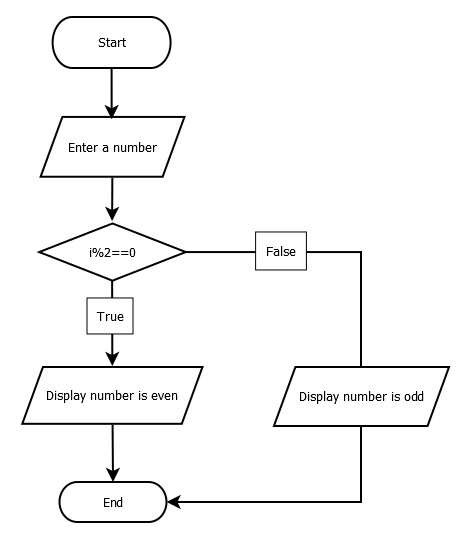# Write a Program to Find Even and Odd Numbers

A number which is divisible by 2 and left 0 remainder is called even number else called odd number. You can refer Algorithm and flowchart of this program as well for better understanding.

#### Flowchart:#### Algorithm:

Begin:

Step1: Take a numeric value from the user

Step2: Get modulus of this number by 2

Step3: if modulus returns 0, number is even

Step4: else number is odd

Step5: Display the result

End:

#### Program Example

This program is tested and executed using Atom IDE with Python 3.8 version. To find even or odd number, we cast user input to int because input function returns string type value.

a = int(input("Enter a Number"))
if(a%2==0):
print("Number is even")
else:
print("Number is odd")
Output:
Enter a Number 20
Number is even
Trending